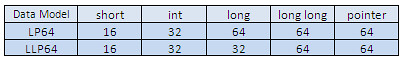# 一個長整數各自表述 (in 64-bit system)

Size of long integer may be different between 64-bit systems (一個長整數各自表述)Many 64-bit compilers today use the LP64 model (including Solaris, AIX, HP, Linux, Mac OS X, and IBM z/OS native compilers). Microsoft’s VC++ compiler uses the LLP64 model.

LLP64 data model 基本上可以說跟 32-bit 的系統一樣，唯一差別只有位址(pointer)改成了 64-bit 而已。資料物件(class, structure) 等如果沒有包含 pointer 的成員的話，整個物件的大小是與 32-bit 系統一樣的！

1. 在 UNIX 下面，long 的大小在 32-bit 與 64-bit 的系統下是不一樣的
2. 同樣是 64-bit 系統，UNIX 與 Windows 對於 long 的大小看法是不一致的

```int8_t     8-bit signed interger
int16_t    16-bit signed interger
int32_t    32-bit signed interger
int64_t    64-bit signed interger
uint8_t    8-bit unsigned interger
uint16_t   16-bit unsigned interger
uint32_t   32-bit unsigned interger
uint64_t   64-bit unsigned interger
```

```INT8       8-bit signed integer
INT16      16-bit signed integer
INT32      32-bit signed integer
INT64      64-bit signed integer
UINT8      8-bit unsigned integer
UINT16     16-bit unsigned integer
UINT32     32-bit unsigned integer
UINT64     64-bit unsigned integer
```

```1 2 3 4 5 6 7 8 9 10 11 12 13 14 #include <stdio.h> #include <sys types.h=""> int main() { printf("sizeof(short) = %d\n", sizeof(short)); printf("sizeof(int) = %d\n", sizeof(int)); printf("sizeof(long) = %d\n", sizeof(long)); printf("sizeof(long long) = %d\n\n", sizeof(long long));   printf("sizeof(size_t) = %d\n", sizeof(size_t)); printf("sizeof(off_t) = %d\n", sizeof(off_t)); printf("sizeof(void *) = %d\n", sizeof(void *)); } </sys></stdio.h>```

## 在〈一個長整數各自表述 (in 64-bit system)〉中有 7 則留言

1. softpapa

stdint.h 是C99 後標準的header，不用分unix 和 windows 吧？

C從來沒要define variable type 的 size ，跟據　c faq 1.1
—————————————————
It’s often incorrectly assumed that C’s types are defined to have certain, exact sizes. In fact, what’s guaranteed is that:

* type char can hold values up to 127;
* types short int and int can hold values up to 32,767; and
* type long int can hold values up to 2,147,483,647.
* something like the relation

sizeof(char) <= sizeof(short) <= sizeof(int) <= sizeof(long) <= sizeof(long long)
————————————————–

版主你的排版很讚，看起來很舒服…:回覆
2. 自動引用通知: [C++]data type – Wuzz